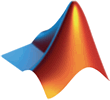# MATLAB入门教程MATLAB 是 Matrix Laboratory（矩阵实验室）的缩写，最初是为了解决线性代数中的矩阵运算问题，后来发展成为算法开发、数据可视化、数据分析、数值计算、信号处理和仿真建模的科学计算语言和编程环境。

MATLAB 的应用领域非常广泛，从事技术研发的理科生基本上都绕不过 MATLAB。

1. MATLAB是什么？
2. MATLAB到底有多厉害？
3. MathWorks公司及其产品介绍
4. MATLAB的组成部分
5. MATLAB R2016b的新增功能
6. MATLAB的应用领域
7. 直接使用MATLAB命令（交互式编程）
8. 一个简单的MATLAB程序（1到100求和）
9. MATLAB R2016b下载、安装及破解教程
10. MATLAB的启动、退出和卸载
11. MATLAB R2016b的目录结构
12. MATLAB R2016b工作界面详解
13. MATLAB R2016b的帮助系统
14. MATLAB的通用命令
15. MATLAB的文件管理
16. MATLAB变量和关键字
17. MATLAB整数类型
18. MATLAB小数（浮点数）类型
19. MATLAB NaN详解
20. MATLAB空数组
21. MATLAB复数类型
22. MATLAB的标点符号
23. MATLAB关系运算符
24. MATLAB ==和～=运算符注意事项
25. MATLAB逻辑运算符
26. MATLAB运算符优先级一览表
27. MATLAB一维数组（向量）的定义
28. MATLAB行向量转置为列向量
29. MATLAB二维数组（矩阵）的创建
30. MATLAB通过下标访问数组元素
31. MATLAB通过下标访问多个数组元素
32. MATLAB通过索引访问数组元素
33. MATLAB布尔法访问数组元素
34. MATLAB数组元素的排序